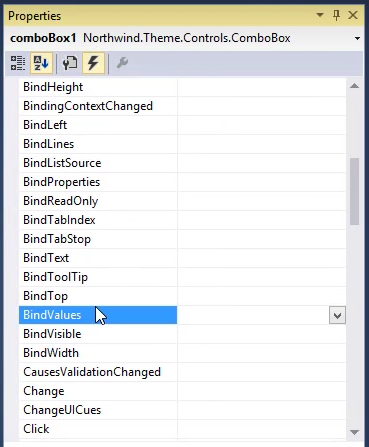﻿ Binding List Control Values to an Expression

# Binding List Control Values to an Expression

In this article we'll bind the ComboBox's `Values` property to an expression that is based on another local column. We'll

• Start by adding a new local Column called "Values" and place a TextBox that is bound to it on the View
``````class DemoComboBox:UIController
{
public TextColumn Values = new TextColumn("Values");
} ``````
• To Bind the `Values` property to an expression, we'll use the `BindValues` event, in the events tab of the property sheet.``````private void comboBox1_BindValues(object sender, StringBindingEventArgs e)
{
e.Value = _controller.Values;
} ``````
• Run the controller and see how when we change the value in the`Values` TextBox, the options of the ComboBox, change accordingly.
• We can also do the same for `DisplayValues`, by adding a local column called `DisplayValues` and then binding the `DisplayValues` property, using the `BindDisplayValues` event.

### Adding the local column to the Controller

``````class DemoComboBox:UIController
{
public TextColumn Values = new TextColumn("Values");
public TextColumn DisplayValues = new TextColumn("Display Values");
} ``````

### Implementing the BindDisplayValues method in the View

``````
private void comboBox1_BindDisplayValues(object sender, StringBindingEventArgs e)
{
e.Value = _controller.DisplayValues;
} ``````